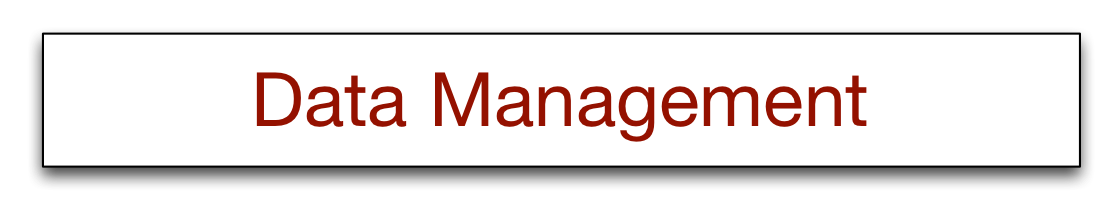# Generating test data

To test the performance of a database, first get some data. There are two alternatives to keying in a large volume of test data. First, existing files can be used as a source of test data and imported into appropriate tables. Second, test data can be generated for each table. Here, we discuss the use of Excel to generate test data. This exercise assumes considerable Excel experience.

## Generating test data with Excel

The RAND function is very useful for generating random test data because it computes an evenly distributed random number r in the range 0 <= r < 1.

### Generating a range of integers

When combined with the ROUND function, RAND can be used to generate random integers. The syntax for round is ROUND(value, digits), where value is the number to round and digits specifies the number of decimal places of the rounding (i.e., ROUND(5.4,0) equals 5). To generate an integer random number between a and b, use: ROUND(RAND()*(b-a) + a, 0)

Thus, to generate quantities sold in the range 1 to 5 use:
ROUND(RAND()*4 + 1,0)

### Generating a range of dates

Excel stores a date as an integer, and days since some starting point are numbered sequentially. Thus to generate random dates in a year, you can add a random integer between 1 and 365 to the last day of the previous year. For example to generate a random date in 1997, enter the following in the designated cells:

 A 1 31-DEC-1996 2 =ROUND(RAND()*364+1,0) + A1

If A2 is not a date, you need to set the format of the cell (Format>Cells ...)

### Randomly selecting text from a list

Imagine that a company has locations in three cities (Atlanta, New York, and Chicago) and sales are distributed, respectively, in the following proportions: .4, .3,.3. To randomly select city names to match this distribution do the following.

• Enter the following data and define A1:B10 as CITY (Insert > Name >Define)

 A B 1 1 Atlanta 2 2 Atlanta 3 3 Atlanta 4 4 Atlanta 5 5 New York 6 6 New York 7 7 New York 8 8 Chicago 9 9 Chicago 10 10 Chicago

• Use RAND with VLOOKUP to select a name from the list.
VLOOKUP(value, table, column) where value is the item to be found in the first column of the array, table is the array, and column is the number of the column to be retrieved the table.
• Using the preceding table VLOOKUP(5, CITY, 2) will return New York
• VLOOKUP(ROUND(RAND()*9+1,0),CITY,2) will return a random city name from the table CITY.

### Randomly selecting a pair of values from a list

Sometimes, you may want to select two values from a list (e.g., the description of an item and its price). VLOOKUP can be used as before. Consider the case where a company sells 10 items and the following price list is established. Here we would name two arrays: ITEM A1:B10 and PRICE B1:C10

 A B C 1 1 Boots - snakeproof 95 2 2 Camel saddle 598 3 3 Compass 8 4 4 Elephant polo stick 352 5 5 Exploring in 10 Easy Lessons 20 6 6 Geo positioning system 295 7 7 Hammock 55 8 8 Hat - polar explorer 34 9 9 How to Win Foreign Friends 26 10 10 Map case 15

To randomly select an item from ITEM, use:

VLOOKUP(ROUND(RAND()*9+1,0),ITEM,2)

Now, another lookup is required to match the selected item to its price. Assume the selected item (e.g., Hammock) is stored in cell X100, then the lookup command would be:

VLOOKUP(X100,PRICE,2)

This would look for the value of X100 (i.e., Hammock) in the first column of PRICE and return its price, which is stored in the second column.

Generate data for the following table:

 Column Values Date In the range 1-Jan-98 to 31-Dec-98 Sales quantity In the range 1 to 8 Location Either Paris, London, or Singapore in the proportions .4,.4, .2 respectively Item One of five possible items (all equally likely) Camera Binoculars Walking boots Backpack Tent Price Respective prices of the above items are: 325 85 156 55 525 Type of sale Catalog or store in a 3:1 ratio

### Hint

It is a good idea to create two worksheets.

1. The data used to generate the test data
2. The test data

### Exercises

1. Generate 100 items (use Edit >Fill) and import the file into your database system and answer the following queries:
• List all sales made during the first quarter of the year
• How many cameras have been sold?
• What is the total value of sales?
2. Generate 1000 items, import the file and repeat the queries. Is there a discernible difference in the time to execute the queries?